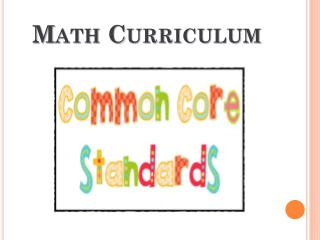Download PresentationMath Curriculum

# Math Curriculum - PowerPoint PPT PresentationDownload Presentation## Math Curriculum

- - - - - - - - - - - - - - - - - - - - - - - - - - - E N D - - - - - - - - - - - - - - - - - - - - - - - - - - -
##### Presentation Transcript

1. Math Curriculum

2. Kindergarten Understand addition as putting together and adding to, and understand subtraction as taking apart and taking from K.OA1 Represent addition and subtraction with objects, fingers, mental images, drawings, sounds (e.g., claps), acting out situations, verbal explanations, expressions, or equations. K.OA2 Solve addition and subtraction word problems, and add and subtract within 10, e.g. by using objects or drawings to represent the problem. K.OA3 Decompose numbers less than or equal to 10 into pairs in more than one way, e.g. by using objects or drawings, and record each decomposition by a drawing or equation (e.g. 5= 2 + 3 and 5 = 4+1) K.OA4 For any number from 1 to 9, find the number that makes 10 when added to the given number, e.g., by using objects or drawings, and record the answer with a drawing or equation. K.OA5 Fluently add and subtract within five Work with numbers 11-19 to gain foundations for place valueK.NBT1 Compose and decompose numbers from 11 to 19 into ten ones and some further ones, e.g., by using objects or drawings, and record each composition or decomposition by a drawing or equation (e.g. 18 = 10 + 8); understand that these numbers are composed of ten ones and one, two, three, four, five, six, seven, eight, or nine ones.

3. What does this look like in Kindergarten? Equations with the = symbol in different positions Using drawings to solve problems Mental Strategies: Number Bonds

4. 1st Grade 1.OA1 Use addition and subtraction within 20 to solve one and two step word problems involving situations of adding to, taking from, putting together, taking apart, and comparing, with unknowns in all positions, e.g. by using drawings and equations with a symbol for the unknown number to represent the problem. 1.OA2 Solve word problems that call for addition of three whole numbers whose sum is less than or equal to 20 1.OA6 Add and subtract within 20, demonstrating fluency for addition and subtraction within 10. 1.OA7 Understand the meaning of the equal sign, and determine if equations involving addition and subtraction are true or false. For example, which of the following equations are true and which are false? 6=6, 7=8-1, 5+2=2+5 , 4+1=5+2 1.OA8 Determine the unknown whole number in an addition or subtraction equation relating to three whole numbers. For example, determine the unknown number that makes the equation true in each of the equations 8+ ? =11, 5 = □ – 3, 6+6 = □

5. 1st Grade continued Use place value understanding and properties of operations to add and subtract1.NBT4 Add within 100, including adding a two-digit number and a one-digit number, and adding a two-digit number and a multiple of 10, using concrete models or drawings and strategies based on place value, properties of operations, and/or the relationship between addition and subtraction; relate the strategy to a written method and explain the reasoning used. Understand that in adding two-digit numbers, one adds tens and tens, ones and ones, and sometimes it is necessary to compose a ten.

6. What does this look like in 1st grade? Adding a 2 digit number and a multiple of 10 bya) drawing Base10 blocksb) making jumps of 10 on an empty number line 45 + 30 = 75

8. 2nd Grade continued 2.NBT7 Add and subtract within 1000, using concrete models or drawings and strategies based on place value, properties of operations, and/or the relationship between addition and subtraction; relate the strategy to a written method. Understand that in adding or subtracting three-digit numbers, one adds or subtracts hundreds and hundreds, tens and tens, ones and ones; and sometimes it is necessary to compose or decompose tens or hundreds. 2.NBT8 Mentally add 10 or 100 to a given number 100-900 and mentally subtract 10 or 100 from a given number 100-900. 2.NBT9 Explain why addition and subtraction strategies work, using place value and the properties of operations.

9. What does this look like in 2nd grade?

10. 3rd Grade 3.NBT2 Fluently add and subtract within 1000 using strategies and algorithms based on place value, properties of operations, and/or the relationship between addition and subtraction.

11. What does this look like in 3rd grade? Decompose into hundreds, tens and ones and recombine (or subtract) with and without regrouping

12. 4th Grade 4.OA3 Solve multistep word problems posed with whole numbers and having whole-number answers using the four operations, including problems in which remainders must be interpreted. Represent these problems using equations with a letter standing for the unknown quantity. Assess the reasonableness of answers using mental computation and estimation strategies including rounding. 4.NBT4 Fluently add and subtract multi-digit whole numbers using the standard algorithm.

13. What does this look like in 4th grade?

14. 5th Grade 5.NBT7 Add, subtract, multiply, and divide decimals to hundredths, using concrete models or drawings and strategies based on place value, properties of operations, and/or the relationship between addition and subtraction, relate the strategy to a written method and explain the reasoning used.

15. What does this look like in 5th grade?

16. Assessments • Rubrics • Checklist • Feedback • Next Steps

17. Common Core Resources for Parents

18. Questions?